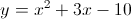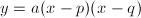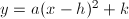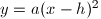# Quadratic Equations, Day 1 of 2

4 teachers like this lesson
Print Lesson

## Objective

SWBAT write quadratic equations in standard, vertex, and factored forms. SWBAT graph quadratic equations efficiently. SWBAT evaluate and solve quadratic equations.

#### Big Idea

Knowledge of quadratic equations is an important prerequisite for studying complex numbers.

## Our Goals

5 minutes

The content of this lesson is first seen in Common Core Algebra 1 and Geometry, but as we transition to the Common Core I found it was necessary to revisit it in my Algebra 2 course to ensure a foundation for our work with complex numbers.

I begin this lesson by writing an easily factorable quadratic equation on the whiteboard, such as.

I then ask the class to tell me what they know about equations like this one.  The students will no doubt recall that it's a quadratic equation, they'll remind me that we can use it to model projectile motion (see What Goes Up) and that it's graph will be a parabola.

I'll respond by laying out the objectives for this lesson (or series of lessons).  First, our aim to to find strategies for efficiently making a graph without technology.  Second, we aim to solve equations of this type under any given circumstances.  Third, we will learn to transform this equation into two other useful forms, factored form and vertex form.

By the time we're finished with this series of lessons, students should have notes that look something like these.

## Learning to Factor

20 minutes

In my experience, students will learn to efficiently factor quadratic equations when two things happen.  First, they need plenty of general explanations of methods and strategies.   Second, they need LOTS of practice.  (Check out this video for some thoughts on this.)

Multiplying binomials is easy as long as you remember the distributive law; there is a process that will lead you to the solution without fail.  Factoring takes patience and judgement as students test one potential solution after another.  Some useful strategies can help them rule out certain options ahead of time.  Experience will give them a "feel" for what is most likely to work.  Along the way, I continually stress the pattern of looking for a pair of number whose product is c and whose sum or difference is b.  (This reminded some of my students of the problems they did on the very first day of class!)

Since factoring a quadratic equation is never strictly necessary, I'm not interested in having them try to factor very complicated expressions.  Instead, my goal is for students to develop the ability to quickly "see" the factors in the simple cases that arise over and over again.  This will strengthen their ability to make use of the structure of quadratic equations (MP 7) and give them greater confidence moving forward.

I've included scans of three practice sets I put together for this purpose.  The problems are chosen so as to grow progressively more difficult, and each one includes a graphing problem to keep those skills fresh.  I assigned one per night for homework while other topics were covered in class.

## Same Equation, Different Form

20 minutes

Having gained experience factoring, it's time to consider the advantages of the factored form of the quadratic equation:.  In this form, the roots of the equation (the x-intercepts) are immediately obvious, but it takes a conversation about factors of zero for most students to see why this is so.  Since my students are now so good at factoring, they can easily write most quadratic equations in factored form.

Next, I remind the class of the vertex form:.  In this form the vertex of the graph, the point (h, k), is clearly visible.  But how do we convert from standard form to vertex form?  By asking ourselves, "What is the nearest perfect square to our equation?"  This means we look for a perfect square,, that would match the first two terms of our equation.  Then we add or subtract a constant to make the two expressions equal.  This is the method of completing the square.

Again, practice makes perfect, so it is important for the class to have the time and opportunity to convert many quadratic equations from standard form into vertex form.  The three practice worksheets introduced in the previous section are a great source of equations for tasking students with practice problems!